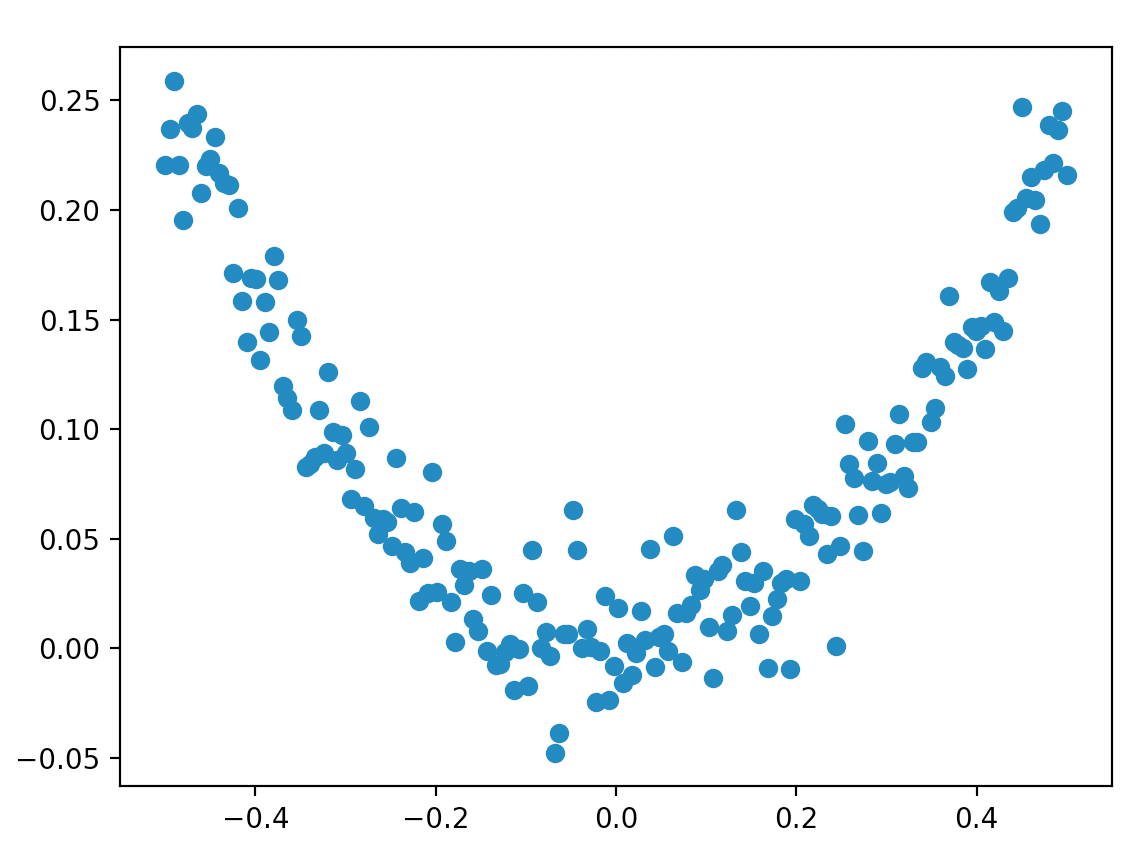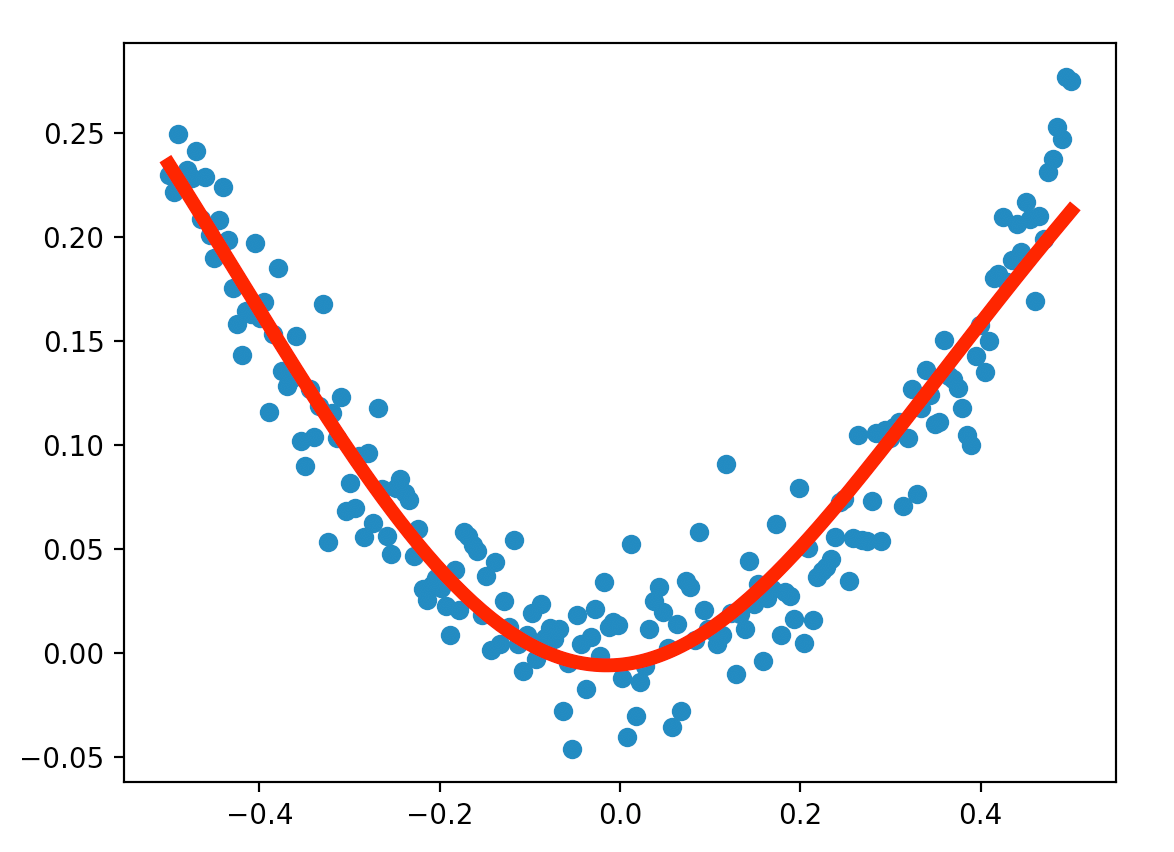# 【Tensorflow基础】第三课：回归模型

Posted by x-jeff on March 7, 2020

# 2.准备训练数据

1
2
3
x_data=np.linspace(-0.5,0.5,200,axis=0).reshape(1,200)
noise=np.random.normal(0,0.02,x_data.shape)
y_data=np.square(x_data)+noise


numpy.linspace在指定的范围内返回均匀间隔的数字。例如，numpy.linspace(0,3,6)可在0到3的范围内均匀生成6个间隔相同的数字：0,0.6,1.2,1.8,2.4,3。因此在上述代码中，我们生成了范围在-0.5到0.5的200个数字作为我们的训练数据。1. numpy.random.rand(d0,d1,...,dn)生成的随机数位于[0,1)之间。参数为生成的随机数的维度。

2. numpy.random.randn(d0,d1,...,dn)生成的随机数来自标准正态分布。参数为生成的随机数的维度。

3. numpy.random.normal(loc,scale,size)生成的随机数来自正态分布。正态分布的$\mu$和$\sigma$由参数locscale传入。

# 3.定义神经网络

## 3.1.网络的输入、输出

1
2
x=tf.placeholder(tf.float32,[1,None])
y=tf.placeholder(tf.float32,[1,None])


[1,None]表示我们的数据只有1行，并且列数不确定，即样本个数不确定。

## 3.2.网络的隐藏层

1
2
3
4
W_L1=tf.Variable(tf.random_normal([10,1]))
b_L1=tf.Variable(tf.zeros([10,1]))
Z_L1=tf.matmul(W_L1,x)+b_L1
A_L1=tf.nn.tanh(Z_L1)


tf.random_normal(shape, mean=0.0, stddev=1.0, dtype=tf.float32, seed=None, name=None)用于生成来自正态分布的随机数，参数说明见下：

• shape：输出的张量形状。
• mean：正态分布的均值，默认为0。
• stddev：正态分布的标准差，默认是1.0。
• dtype：输出的类型，默认是tf.float32
• seed：随机数种子，是一个整数，当设置之后，每次生成的随机数都一样。
• name：操作的名称。

## 3.3.网络的输出层

1
2
3
4
W_L2=tf.Variable(tf.random_normal([1,10]))
b_L2=tf.Variable(tf.zeros(1,1))
Z_L2=tf.matmul(W_L2,A_L1)+b_L2
prediction=tf.nn.tanh(Z_L2)


# 4.cost function

1
loss=tf.reduce_mean(tf.square(y-prediction))


tf.reduce_mean函数用于计算张量tensor沿着指定的数轴（tensor的某一维度）上的平均值，主要用作降维或者计算tensor的平均值。

tf.reduce_mean如果不指定轴（axis），则计算所有元素的均值。

# 5.优化方法

1


# 6.运行网络

1
2
for _ in range(2000):
sess.run(train,feed_dict={x:x_data,y:y_data})


⚠️记得通过feed操作喂入训练数据以及其标签。# 7.代码地址